Catching the Wind

Introduction

The wind can blow papers, tussle hair, and even turn umbrellas inside out. The power of wind is clear, but it is useless unless we can convert a breeze into a more practical form. Humans have done just that, harnessing wind power using sailboats, kites, and, most recently, wind turbines. Wind turbines are frequent sights on hillsides, with their familiar and canonical shape: a tall white tower with three long, narrow blades. However, why are current windmills designed in this way? Imagine the Dutch windmills with four large and flat blades, or the small metallic windmills on farms used to raise water for irrigation. There are even designs, such as the Savonius wind turbine, that look like egg-beaters and spin vertically .

The variation in designs reflects the variation of purposes and environments. The white wind turbines are designed to provide a maximum of electrical power output. In the Dutch mills, the only energy needed was that to grind wheat. Cost, as well, determines the size and weight of the individual turbine. However, physics does dictate some universal laws and constraints that hold true for all designs. The best example of this is Betz's limit, which dictates the maximum efficiency of a windmill . Just as Carnot's engine provides a universal statement independent of the design of an engine, Betz's Law holds for any windmill.

In this article, we will analyze several of the factors that go into the current wind turbine design. Beginning with a quantitative analysis of the wind's power, we then turn to a look at Betz's formula for the power of a wind turbine, and finally the effect that the number of blades has on the turbine.

Air Dynamics

A windmill can be approximated as a 'black box,' as shown in Figure 1. Wind comes in from the left, passes through the turbine, and exits at the right. The way that a windmill gets power is by slowing down the passing air, so we imagine the simple case where there are two distinct air velocities to the left and right of the windmill. If the final wind speed is the same as the initial, then the windmill has extracted no energy from the wind. On the other hand, imagine the final wind speed is zero. In that case, the windmill is basically a wall, stopping all the wind. If we desired a sail for a boat, this would be great. However, with no wind going past the turbine, it turns out we will not create the most efficient windmill. To analyze the situation more closely, we need to determine how much power a windmill actually removes from the air.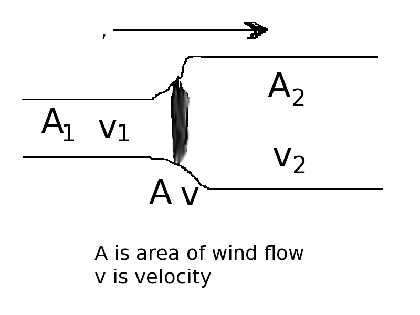Fig. 1:Generic wind flow around a Windmill. The incoming column of air from the left passes through the windmill (shown in the middle), decreasing the air speed and increasing the size of the air column. It is this reduction in air speed which gives power to the windmill.

To find the formulas governing the efficiency of the windmill shown in Figure 1, we need to outline the formalism of energy and power in fluid flows, following the discussion given by Ragheb . To begin with, the energy of a mass of moving air is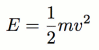This is simply familiar kinetic energy. The power is just the derivative of energy with respect to time. This means, for instance, that the power in a column of air of cross-section A, velocity v, and density ρ is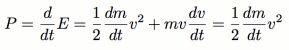where we used the fact that the velocity in the stream is constant. To find dm/dt, we imagine a column of air of cross-section area A moving along. We find dm/dt by determining how much air passes through the area in a given time.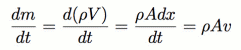Thus we find that the power is given byBetz's Limit

Now that we have the power of a free-flowing wind stream, we turn to the question of how much power is extracted by our windmill in Figure 1. We must be careful here, because the answer is not simply the power equation stated above. Following Ragheb's anaysis , to find the power extracted, we find the energy difference in the two sides of the windmill and take the derivative with respect to time. This gives us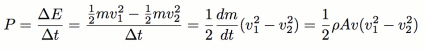This expression tells us the power extracted by our windmill. In the first step, we simply rewrote the energy difference on both sides of the mill. To find the dm/dt, we compute the mass passing by the windmill (of area A) per time. Note that we have reasonably assumed the density of the air is the same on both sides. Also note that the cross section on the right side of the windmill is larger than on the left side. This simply reflects the fact that our air column will be wider when it is slower. This is clear since, as mentioned, the density will not change. Thus, to avoid a build up of air mass behind the windmill, the width of the stream must increase.

At this point, it is useful to write v, the wind velocity directly at the windmill, in terms of v1 and v2. The naive guess would be that v=1/2(v1+v2), since the speed at the interface could reasonably be treated as the average of the speeds on either side. Under closer investigation, see , this is in fact true. This means we end up with the extracted power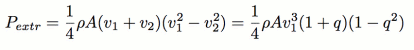where we define q as v2/v1. To simplify this further, consider the ratio of the extracted power to the initial power in the column of undisturbed air. This is known as the efficiency of a windmill.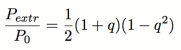This function is plotted in Figure 2. As we noted before, when q=1, the wind passes through unaltered, and the power output will be zero. When q=0, we get the case that the windmill stops all the air, and as we discussed the power here is not the highest possible. To find the peak of the graph, one spends a few minutes optimizing Pextr/P0 with respect to q, and finds the (physical) solution that q=1/3 gives the maximum. This means the best windmill is one where the final wind speed is one third of the initial wind speed. This is a surprisingly simple answer.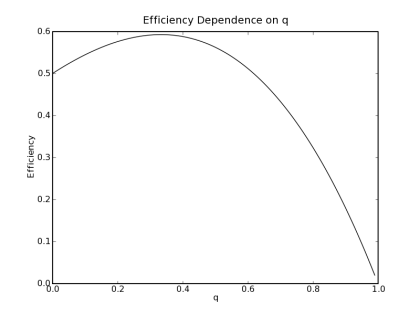Fig. 2: Plot of Windmill Efficiency vs. Wind Speed Ratio. The variable q represents the ratio of initial wind speed to final wind speed. For a certain value of q, the efficiency of a windmill is maximized.

Plugging q=1/3 into the above equations, we find that the maximum efficiency Pextr/P0 is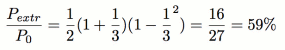This is known as Betz's limit. It means the maximum possible efficiency of a windmill is simply and concretely 59%. In thermodynamics, the Carnot engine sets the theoretical maximum for efficiency. In this case, we have reached a similar universal statement. In reality though, just as a Carnot cycle does not make a good engine for your car, it is impractical to create a windmill with this efficiency. Instead, most windmills operate at efficiencies of roughly 40%, as noted by Ragheb . This falls well under the universal limit we found using just simple equations.

Maximizing Power with Betz's Formula

Now that we know the maximum efficiency for a windmill, we can abandon the "black box" idea and begin looking at specific designs. We narrow our analysis to the horizontal-axis turbines, the most common variety. What is the best design for such a wind turbine? For example, we can imagine varying the radius of the turbine, the height of the tower, and the number of blades. What optimization rules have led to the canonical design? Using the analysis of the previous section, we can write the formula governing power-output of an ideal windmill.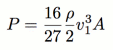This is known as Betz's formula , and is the maximum theoretically allowed power output, using Betz's limit. We see that the power output depends on three factors: the air density, the wind speed, and the area swept out by the turbine. The first of these is not something we can vary as we design out windmill, so we focus on optimizing the final two variables.

That the power depends upon the wind velocity is no surprise. In stronger wind, windmills spin faster. However, the strength of this relation is at first surprising. The power depends upon the cube of the wind speed. Thus, we see the most important factor in power output is finding a location with strong winds . This is in fact done, and is why windmills are generally found along ridges instead of deep in forests.

However, we can do more to optimize wind speed than just the placement of the windmill. The height of our windmill is an important component. As discussed in Ragheb , the wind speed is a function of height. Starting from a vanishing wind speed at the ground, the wind speed increases as one goes higher. This is familiar to those who have stood atop a tall tower. This dependence can be approximated by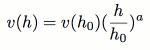In this equation, we find the wind speed based on comparison with the known wind speed at a height h0. The constant a depends on the environment, but is on the order of .1 . Thus, the velocity increases slowly with increasing height. Doubling the height here would result only in a roughly 10% increase in speed. However, with the cubic dependence on wind speed in Betz's formula, the modest gain in wind speed results in a significant increase in power output.

Thus, placing the wind turbines high above the ground leads to an increase in power. This is one reason that windmills are placed on tall towers. A windmill stationed low to the ground would be ineffective. Just looking at Betz's formula, we see that, in fact, the higher the windmill the better. This is, of course, not true due to practical constraints. At a certain height, it becomes difficult or uneconomical to build taller windmills.

We now turn to the final variable in Betz's formula, which is the area of the turbine. This is merely given by πR2, where R is the length of the blades on the windmill. An increase in the length of the blades will lead to a quadratic increase in the power output. As it turns out, the power output actually depends more sensitively on the wind speed than the turbine radius, which may be surprising. Again, though naive optimization using Betz's formula would lead us to thinking the bigger the turbine the better, practical and monetary considerations interfere with this conclusion. For instance, at the very least, we do not want the blades reaching down to the ground.

The blades of a windmill are perhaps the most studied part of the turbine design, and are well summarized by Adkins and Liebek  and Jischke . For instance, the blade design is closely related to the design of propellors on aircraft. However, we will focus on a simpler component of blade-design: the number of blades. In the current wind turbines used for generating electricity, there are three, and always three blades. But, why? The old Dutch mills had four blades, and the small metal farmer mills have roughly a dozen blades.

As outlined in , there are several considerations used when deciding how many blades a turbine should have. The first is in the efficiency of the turbine. A very large number of blades would decrease the efficiency of a turbine. Intuitively this is clear. The drag caused by 100 spinning blades would be much greater than that caused by 3. In trying to find the optimum number of blades, we are led to choose a low number.

However, there is a second consideration, which is unrelated to efficiency. Should a turbine have an odd or even number of blades? At first, it may not seem to matter much whether there are two blades or three. However, upon further inspection , a simple difference is revealed. The balance and stability of a windmill greatly depends on the number of blades.

First, let us consider a windmill with two blades. Imagine the instant that the blades are vertical. At that moment, the bottom blade is passing in front of the tower, drastically cutting down the wind force on the blade. On the other hand, the top blade is fully exposed to the maximum wind speed - recall that wind speed increases with height. There is a greater force on the top blade than the bottom blade. The net effect is that there is a torque on the turbine. It has a tendency to be tilted backwards slightly. This wobble leads to an undesired instability in the windmill, and leads to engineering headaches when it comes to designing the hub of the windmill.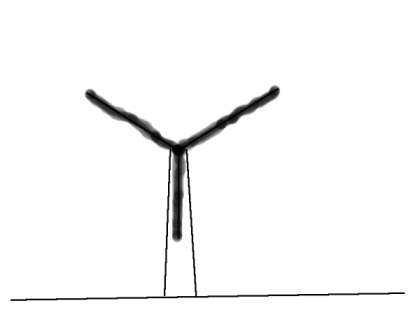Fig. 3: Windmill with Three Blades. As the lower blade passes the tower, the other blades will be getting more force from the wind. This causes a backwards torque, but with an odd number of blades this torque is considerably less than with an even number.

Now consider a windmill with three blades. In this case, when one of the blades passes the tower, the other two are each at 30° above the horizontal. They do indeed get more force from the wind than the lower blade. However, this situation is better than the two-blade case for two reasons. First, since the blades are not at their zenith, the force on each is not at a maximum. Secondly, most of the forces cancel between these two blades, since they are nearly opposite one another. Only a fraction of the force is directed at tilting the turbine "backwards." This case is far more stable than the two-blade case.

Thus we see that, in general, an odd number of blades is easier to deal with when designing a windmill. There will be a less pronounced wobble than in cases where there are an even number of blades.

Conclusion

By looking at the physics behind windmills, we now have a better understanding of why windmills look the way they do. Current windmills are first and foremost power plants. From Betz's formula, we find the important variables affecting power output. The wind speed is the most important factor, and this is optimized both by choosing good locations and by placing the turbines on tall towers. We see that the length of the blades is related to the power output; specifically the power is proportional to the square of the radius. This, too, can be optimized in designing windmills. Finally, the number of blades is an important design consideration, if not for the efficiency, but for the stability of the windmill.

The increasing concerns over climate change and environmental awareness will increasingly make wind power an appealing source of energy. More and more windmills will pop up on hillsides, and it is important to know the physics behind their design. Windmills do not have an arbitrary design, but rather a design dictated by the physics of wind power.

© 2007 Andy Hall. The author grants permission to copy, distribute and display this work in unaltered form, with attribution to the author, for noncommercial purposes only. All other rights, including commercial rights, are reserved to the author.

References

 T. Suzuki, H. Okitsu. "Characteristics of Savonius Windmill Power System with a Synchronous Generator." Wind Engineering 6, 131 (1982).

 M. Ragheb, "Energy and Power Content of the Wind" http://netfiles.uiuc.edu/mragheb, 2007.

 M. Ragheb, "Theory of Wind Machines, Betz Equation" http://netfiles.uiuc.edu/mragheb, 2007.

 C. Adkins and R. Liebeck. "Design of Optimum Propellers," J. Propul. Pwr, 10, No. 5, Sept 1994.

 M. Jischke, "On the Aerodynamics of Windmill Blades," Proc. Okla. Acad. Sci. 56 121 (1976).

 Z. Salameh. "Optimum Windmill-Site Matching," IEEE Trans. Energ. Conv. 7, No. 4, 1992.

 Danish Wind Industry Association, "Wind Turbines: How many blades?" http://www.windpower.org/en/tour/design/concepts.htm, 2003.